# Cube Roots and Perfect Cubes Lesson Plan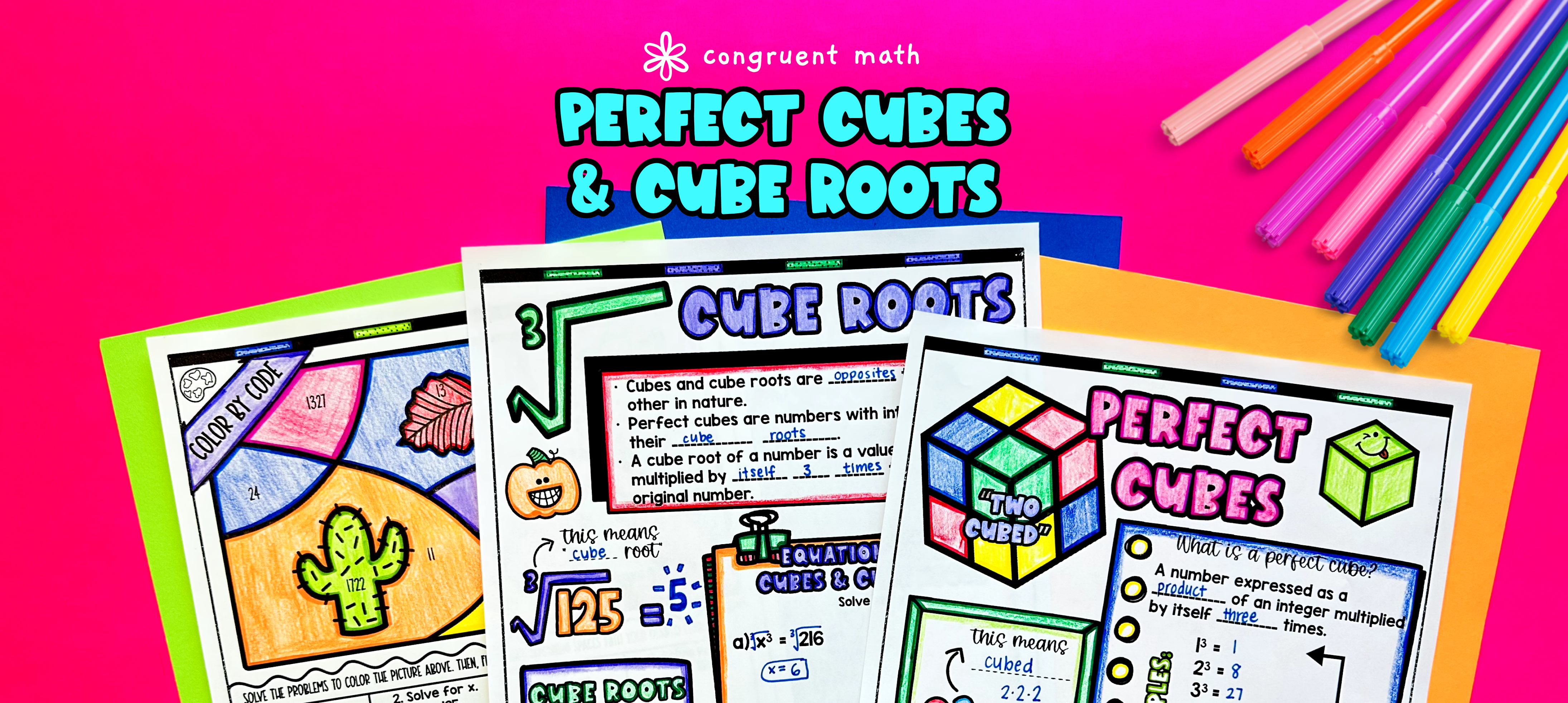Pin This

## Overview

Ever wondered how to teach cube roots and perfect cubes in an engaging way to your eighth-grade students?

In this lesson plan, students will learn about cube roots and perfect cubes and their real-life applications. Through artistic, interactive guided notes,a maze worksheet, and a color by code activity students will gain a comprehensive understanding of cube roots and perfect cubes.

The lesson culminates with a real-life example that explores how this math concept is applied to designing water tanks.

## Get the Lesson Materials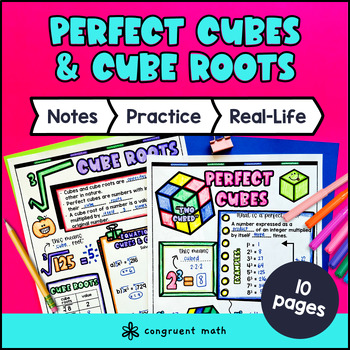\$4.25

## Learning Objectives

After this lesson, students will be able to:

• Define perfect cubes and cube roots.

• Identify perfect cubes and solve simple equations in the form x³ = p.

• Apply the concept of perfect cubes and cube roots to solve real-world problems.

## Prerequisites

Before this lesson, students should be familiar with:

• Basic multiplication and division skills

• Understanding of the concept of exponents and their rules

• Familiarity with the concept of square roots and finding square roots of numbers

• Knowledge of rational numbers and their operations

## Key Vocabulary

• Perfect cubes

• Cube roots

• Equations

• Rational numbers

## Procedure

### Introduction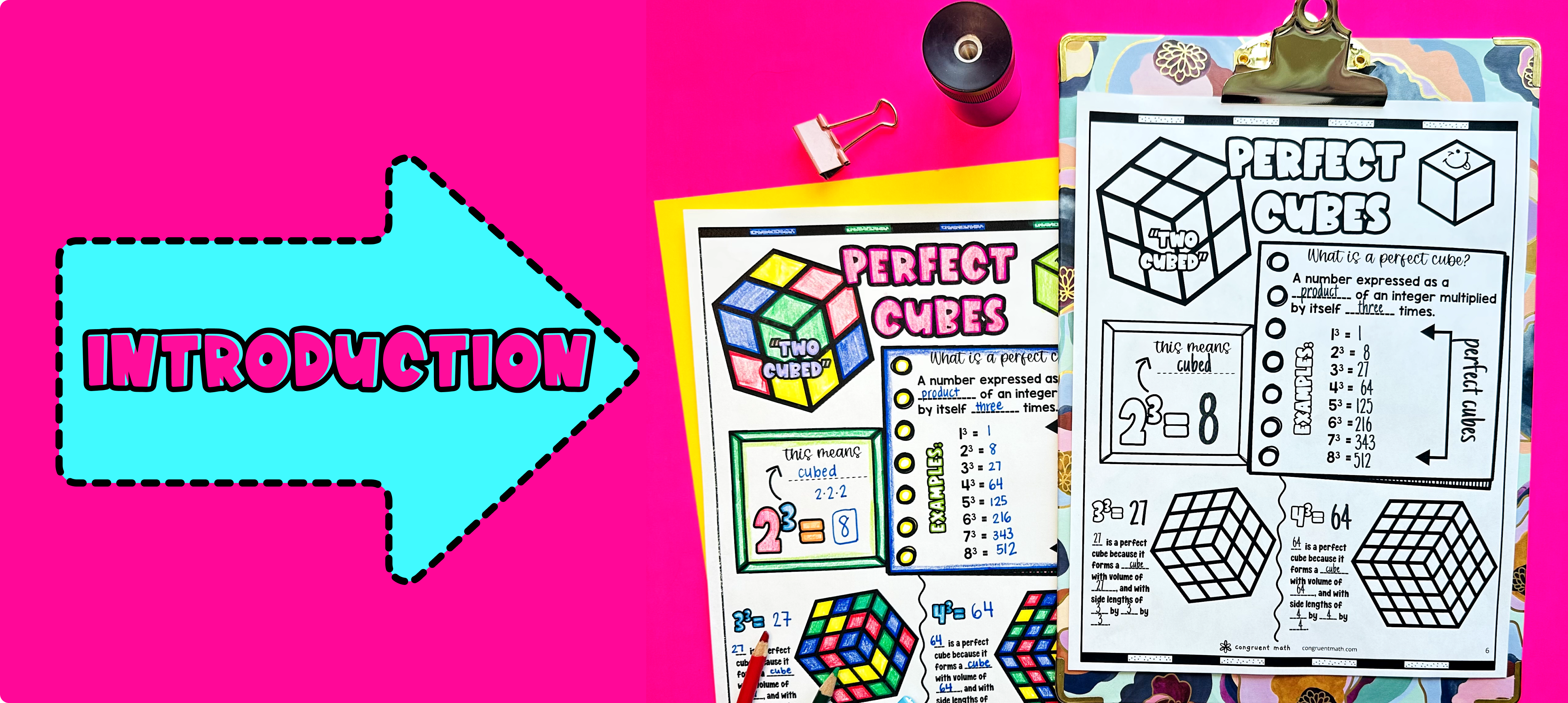Pin This

As a hook, ask students why understanding cube roots and perfect cubes is important in real life. Refer to the real-life application on the last page of the guided notes as well as the FAQs below for ideas.

Use the first page of the guided notes to introduce perfect cubes. Walk through the concept of perfect cubes and explain that a perfect cube is the result of multiplying a number by itself three times. Emphasize key points such as how to recognize perfect cubes and how to express perfect cubes using exponents. Refer to the FAQ below for ideas on how to respond to common student questions.

Use the second page of the guided notes to introduce cube roots. Explain that a cube root is the opposite operation of finding a perfect cube and is the number that, when multiplied by itself three times, equals a given value. Walk through the process of finding cube roots using the ∛ symbol and how to express cube roots using exponents. Refer to the FAQ below for ideas on how to respond to common student questions.

If your class has a wide range of proficiency levels, you can pull out students for reteaching, and have more advanced students begin work on the practice exercises.

### Practice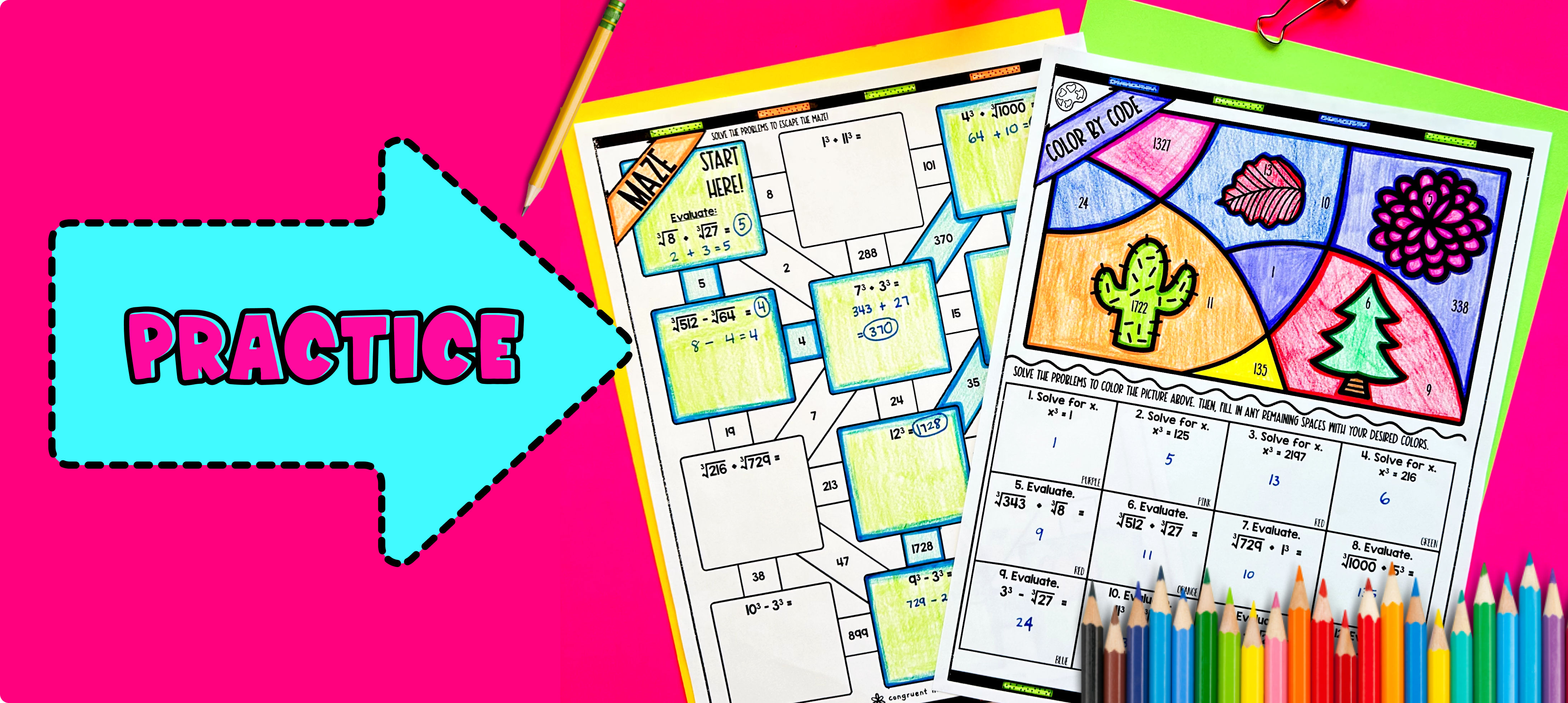Pin This

Have students practice perfect cubes and cube roots using the practice maze activity provided in the resource. Walk around the classroom to answer any student questions and provide support as needed.

Fast finishers can dive into the color by code activity for extra practice. You can also assign these activities as homework for the remainder of the class.

### Real-Life Application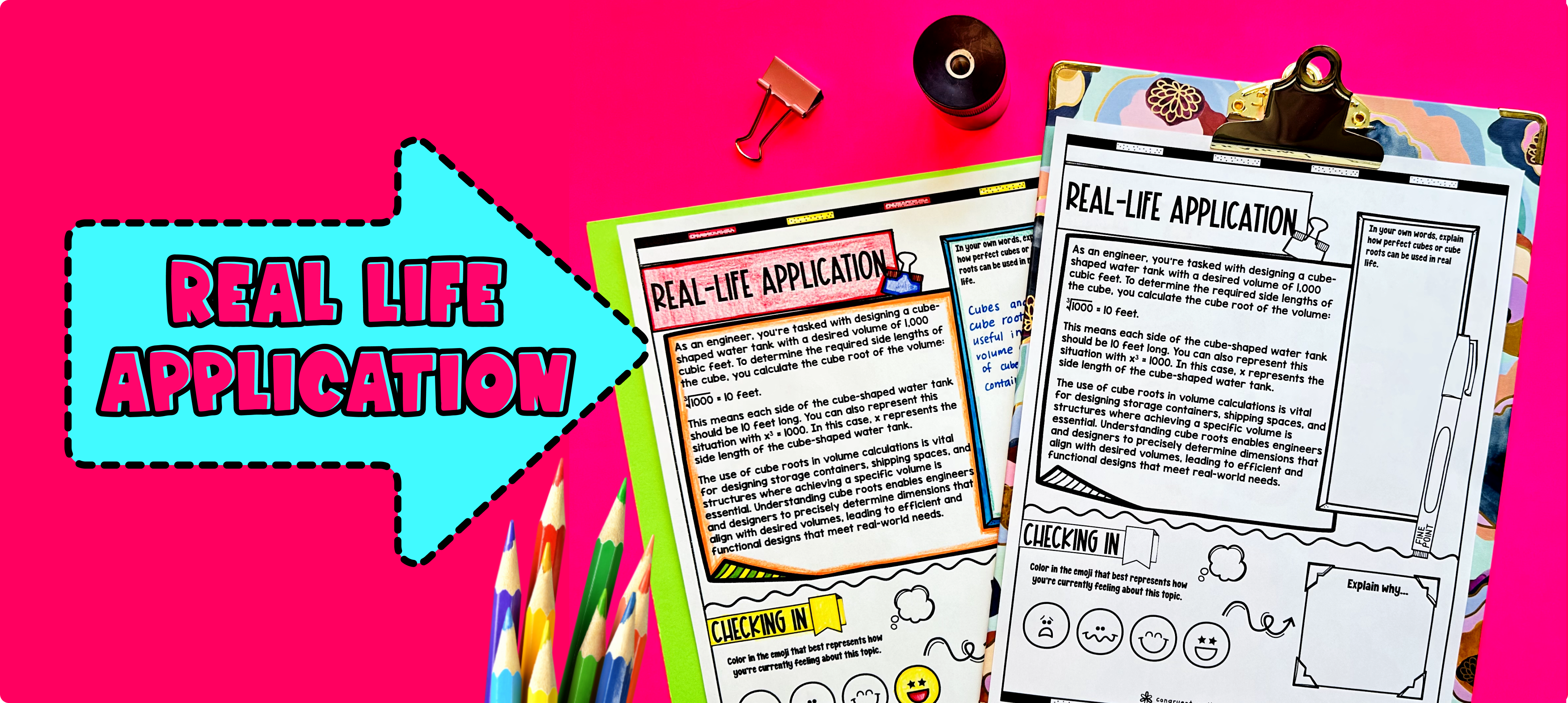Pin This

Bring the class back together, and introduce the concept of designing cube-shaped water tanks as a real-world application of perfect cubes and cube roots. In this application, students will learn how to calculate the dimensions of a water tank based on its capacity.

Explain to the students that water tanks are commonly used to store and supply water in various settings, such as residential areas, farms, and industrial sites. The capacity of a water tank is measured in cubic units, which is where the concept of perfect cubes and cube roots becomes relevant.

Using the example of a cubic water tank, discuss with the students the formula for calculating the volume of a cube: V = x^3. Explain that in this formula, the side length (x) is the only dimension needed to determine its capacity.

Then guide a discussion on other places in everyday life that use perfect cubes in everyday life. Students can use the space in the guided notes to brainstorm their response first before sharing with the class.

Refer to the FAQ section for more ideas on how to further explore the real-life application of perfect cubes and cube roots, such as exploring different shapes of water tanks or discussing the importance of precise calculations in engineering and construction projects.